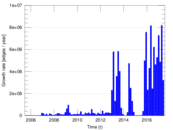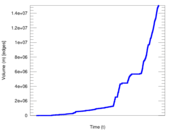# Wikipedia edits (ceb)

This is the bipartite edit network of the Cebuano Wikipedia. It contains users and pages from the Cebuano Wikipedia, connected by edit events. Each edge represents an edit. The dataset includes the timestamp of each edit.

 Code `ceb` Internal name `edit-cebwiki` Name Wikipedia edits (ceb) Data source http://dumps.wikimedia.org/ AvailabilityDataset is available for download Consistency checkDataset passed all tests Category Authorship network Dataset timestamp 2017-10-20 Node meaning User, article Edge meaning Edit Network formatBipartite, undirected Edge typeUnweighted, multiple edges Temporal dataEdges are annotated with timestamps

## Statistics

 Size n = 8,486,200 Left size n1 = 3,132 Right size n2 = 8,483,068 Volume m = 15,072,104 Unique edge count m̿ = 11,792,890 Wedge count s = 37,976,418,248,406 Claw count z = 1.013 69 × 1020 Cross count x = 2.105 84 × 1026 Maximum degree dmax = 10,915,796 Maximum left degree d1max = 10,915,796 Maximum right degree d2max = 29,339 Average degree d = 3.552 14 Average left degree d1 = 4,812.29 Average right degree d2 = 1.776 73 Average edge multiplicity m̃ = 1.278 07 Size of LCC N = 8,484,928 Diameter δ = 13 50-Percentile effective diameter δ0.5 = 1.514 79 90-Percentile effective diameter δ0.9 = 1.926 63 Median distance δM = 2 Mean distance δm = 2.067 60 Gini coefficient G = 0.666 327 Balanced inequality ratio P = 0.248 930 Left balanced inequality ratio P1 = 0.006 225 41 Right balanced inequality ratio P2 = 0.368 405 Power law exponent γ = 5.307 60 Tail power law exponent γt = 5.911 00 Degree assortativity ρ = −0.507 473 Degree assortativity p-value pρ = 0.000 00 Spectral norm α = 41,497.9 Spectral separation |λ1[A] / λ2[A]| = 17.435 2

## Plots

### Degree distribution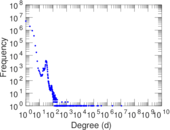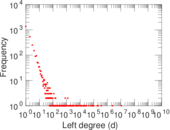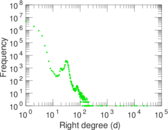### Cumulative degree distribution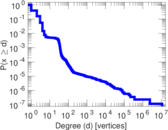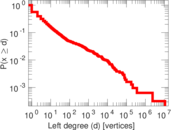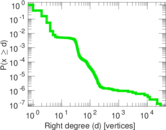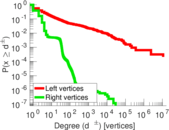### Spectral distribution of the adjacency matrix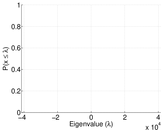### Spectral distribution of the normalized adjacency matrix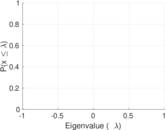### Spectral distribution of the Laplacian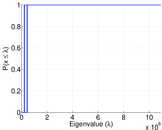### Spectral graph drawing based on the adjacency matrix### Spectral graph drawing based on the Laplacian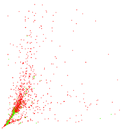### Spectral graph drawing based on the normalized adjacency matrix### Hop distribution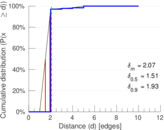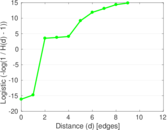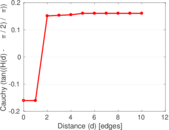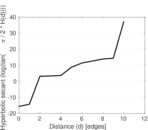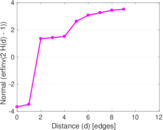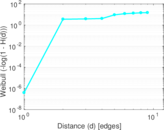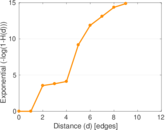### Temporal distribution